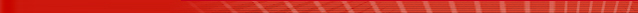## Equational Theorem Proving

Mathematica 6 for the first time brings general automated theorem proving into an immediate interactive environment. Extending Mathematica's already uniquely powerful algebraic theorem-proving capabilities, Mathematica 6 introduces equational theorem proving capable of operating on industrial-scale arbitrary abstract systems of axioms or relations, and integrating theorem proving into the computational workflow.
• Advanced equational theorem proving automatically accessed directly from FullSimplify.
• Full support for ForAll, Exists, etc. quantifiers.
• Immediately allows Mathematica arbitrary symbolic notation for maximum readability.
• Uses state-of-the-art unfailing completion methods.

Related Functions